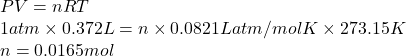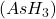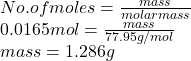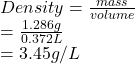## PLZ HELP *NO LINKS* 1) How many moles of gaseous arsine (AsH3) occupy 0.372 L at STP? In sig fig 4 2) What is the density

Question

PLZ HELP *NO LINKS*
1) How many moles of gaseous arsine (AsH3) occupy 0.372 L at STP?
In sig fig 4
2) What is the density of gaseous arsine?
In sig fig 4
Thanks!

in progress 0
5 months 2021-08-24T04:57:18+00:00 1 Answers 40 views 0

## Answers ( )

1. Answer: (1). There are  0.0165 moles of gaseous arsine (AsH3) occupy 0.372 L at STP.

(2). The density of gaseous arsine is 3.45 g/L.

Explanation:

1). At STP the pressure is 1 atm and temperature is 273.15 K. So, using the ideal gas equation number of moles are calculated as follows.

PV = nRT

where,

P = pressure

V = volume

n = number of moles

R = gas constant = 0.0821 L atm/mol K

T = temperature

Substitute the values into above formula as follows.2). As number of moles are also equal to mass of a substance divided by its molar mass.

So, number of moles of Arsine(molar mass = 77.95 g/mol) is as follows.Density is the mass of substance divided by its volume. Hence, density of arsine is calculated as follows.Thus, we can conclude that 0.0165 moles of gaseous arsine (AsH3) occupy 0.372 L at STP and the density of gaseous arsine is 3.45 g/L.